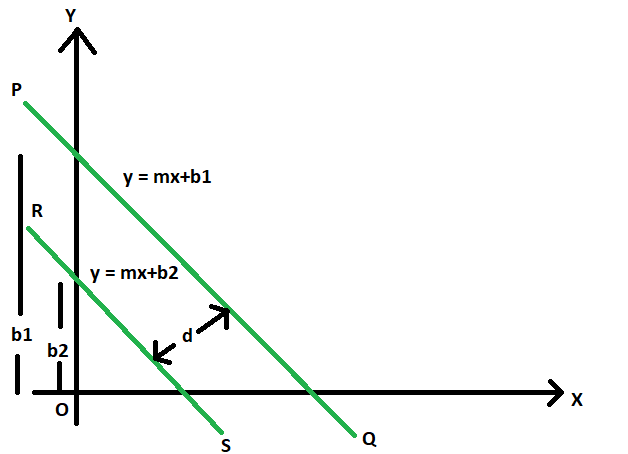Open in App
Not now

# Distance between two parallel lines

• Last Updated : 07 Jun, 2022

Given are two parallel straight lines with slope m, and different y-intercepts b1 & b2.The task is to find the distance between these two parallel lines.
Examples:

Input: m = 2, b1 = 4, b2 = 3
Output: 0.333333

Input: m = -4, b1 = 11, b2 = 23
Output: 0.8Approach:

1. Let PQ and RS be the parallel lines, with equations
y = mx + b1
y = mx + b2

2. The distance between these two lines is the distance between the two intersection points of these lines with the perpendicular line.Let that distance be d

3. So, equation of the line perpendicular to PQ and RS can be
y = -x/m

4. Now, solving the perpendicular line with PQ and RS separately to get the intersecting points (x1, y1) & (x2, y2), we get,

5. From PQ
y = mx + b1
y = -x/m
(x1, y1) = ( -b1*m/(m^2 + 1), b1/(m^2 + 1))

6. From RS
y = mx + b2
y = -x/m
(x2, y2) = ( -b2*m/(m^2 + 1), b2/(m^2 + 1))

7. So, d = distance between (x1, y1) and (x2, y2)Below is the implementation of the above approach

## C++

 // C++ program find the distance // between two parallel lines   #include  using namespace std;   // Function to find the distance  // between parallel lines  double dist(double m, double b1, double b2) {     double d = fabs(b2 - b1) / ((m * m) - 1);     return d; }   // Driver Code int main() {     double m = 2, b1 = 4, b2 = 3;     cout << dist(m, b1, b2);     return 0; }

## Java

 // Java program find the distance // between two parallel lines class GFG {       // Function to find the distance  // between parallel lines  static double dist(double m,                  double b1, double b2) {     double d = Math.abs(b2 - b1) /                      ((m * m) - 1);     return d; }   // Driver Code public static void main(String[] args) {     double m = 2, b1 = 4, b2 = 3;      System.out.println(dist(m, b1, b2)); } }   // This code is contributed by Code_Mech.

## Python3

 # Python3 program find the distance # between two parallel lines   # Function to find the distance  # between parallel lines  def dist(m, b1, b2):     d = abs(b2 - b1) / ((m * m) - 1);     return d;   # Driver Code def main():     m, b1, b2 =2,4, 3;     print(dist(m, b1, b2)); if __name__ == '__main__':     main()   # This code contributed by PrinciRaj1992

## C#

 // C# program find the distance // between two parallel lines using System;   class GFG {       // Function to find the distance  // between parallel lines  static double dist(double m,                     double b1, double b2) {     double d = Math.Abs(b2 - b1) /                        ((m * m) - 1);     return d; }   // Driver Code public static void Main() {     double m = 2, b1 = 4, b2 = 3;     Console.Write(dist(m, b1, b2)); } }   // This code is contributed by Akanksha Rai

## PHP

 

## Javascript

 

Output:

0.333333

Time Complexity: O(1)

Auxiliary Space: O(1)

My Personal Notes arrow_drop_up
Related Articles